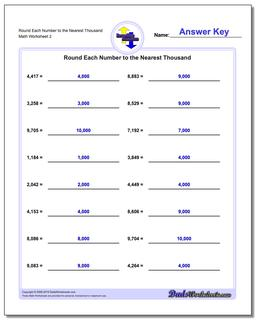# Math Worksheets: Rounding Numbers: Rounding Numbers: Round Each Number to the Nearest Thousand (Second Worksheet)## Round Each Number to the Nearest Thousand (Second Worksheet)

PropertyValue
DescriptionRound Each Number to the Nearest Thousand: Rounding worksheets where whole numbers must be rounded to the same place value as the most significant digit. This is a great starting place for building rounding concepts. (Second Worksheet)
Resource TypeWorksheet